Question

# Consider the following UNBALANCED equation: Na(s) + Cl2(g) -> NaCl(s). a.) Calculate the limiting reagent when...

Consider the following UNBALANCED equation: Na(s) + Cl2(g) -> NaCl(s).

a.) Calculate the limiting reagent when 55.0 g of react with 67.2 g of Cl2. (Show all work, and clearly state which is the limiting reagent.)

b.) What is the theoretical yield, in grams, of NaCl?

c.) If 105g of NaCl is produced from the reaction, what is the percent yield of NaCl?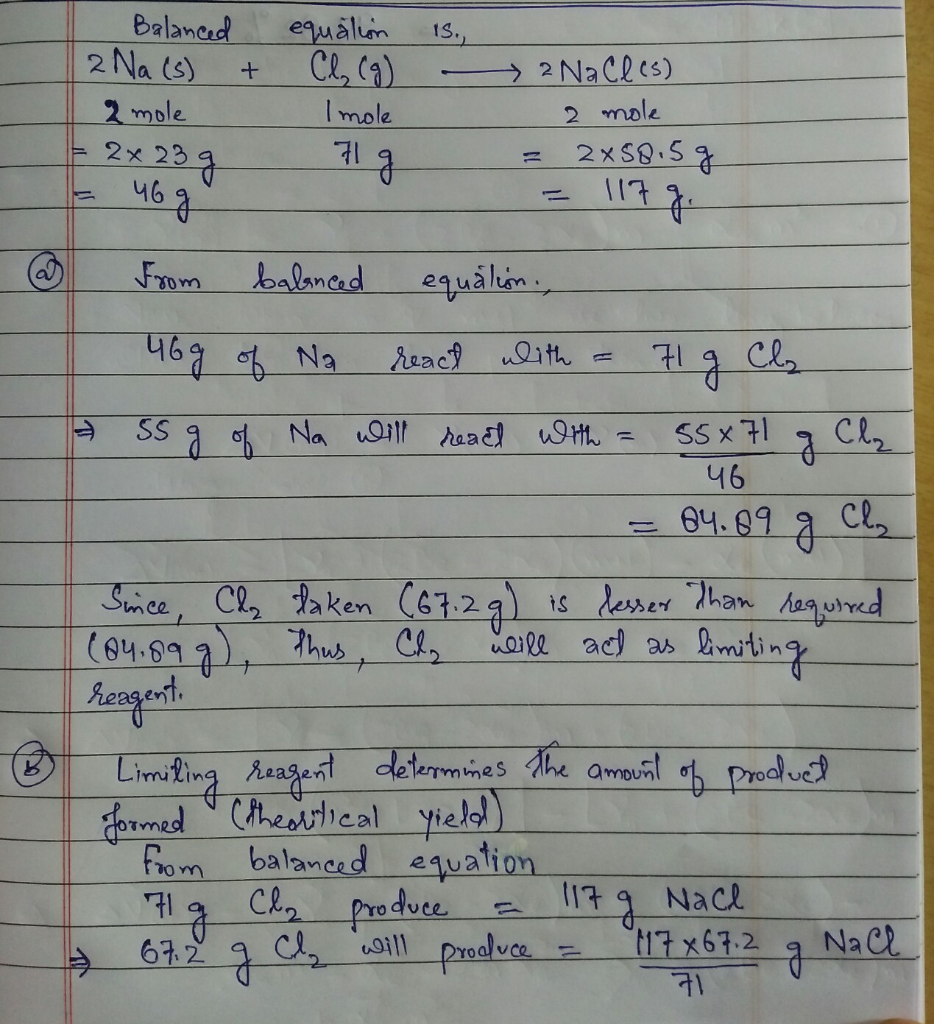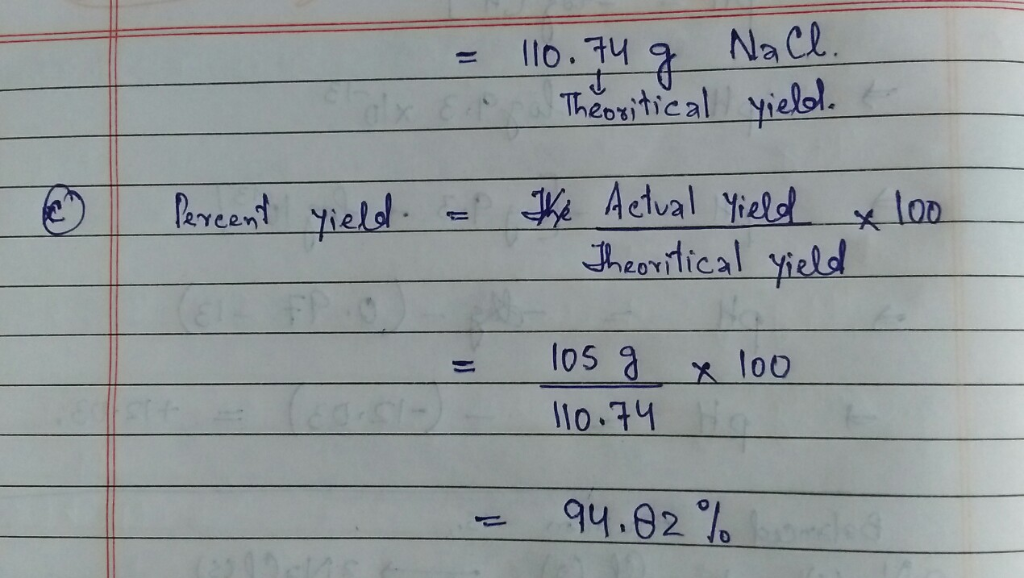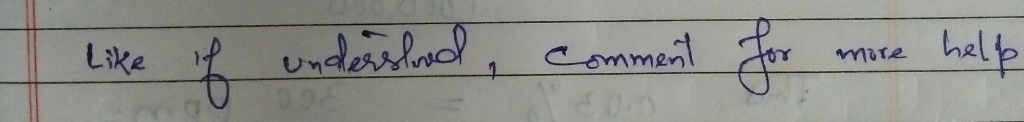#### Earn Coins

Coins can be redeemed for fabulous gifts.

Similar Homework Help Questions
• ### 3. Consider the following UNBALANCED equation: Na (s) Cl2(g) NaCl (s) + → a. Calculate the...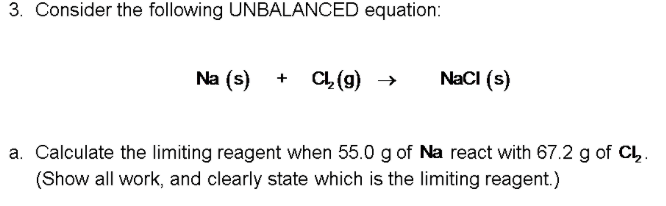3. Consider the following UNBALANCED equation: Na (s) Cl2(g) NaCl (s) + → a. Calculate the limiting reagent when 55.0 g of Na react with 67.2 g of CL, (Show all work, and clearly state which is the limiting reagent.)

• ### Q2. Sodium and chlorine react to form sodium chloride: 2 NaCl(s) 2 Na(s) + Cl2(8) What...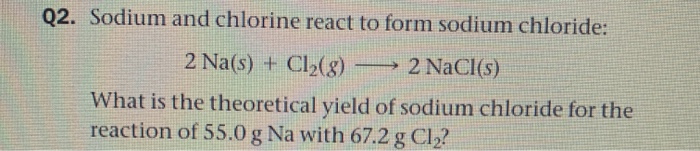Q2. Sodium and chlorine react to form sodium chloride: 2 NaCl(s) 2 Na(s) + Cl2(8) What is the theoretical yield of sodium chloride for the reaction of 55.0 g Na with 67.2 g Cl2?

• ### 1. Consider the reaction: MnO2 (s) + 4 HCl (g)MnCl2 (s) + Cl2 (g) + 2 H20 (g) If 10.00 g of MnO2 ...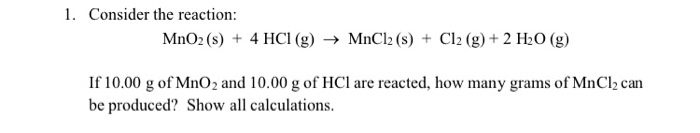1. Consider the reaction: MnO2 (s) + 4 HCl (g)MnCl2 (s) + Cl2 (g) + 2 H20 (g) If 10.00 g of MnO2 and 10.00 g of HCl are reacted, how many grams of MnCl2 can be produced? Show all calculations 2. Consider the following unbalanced equation: NH4NOs(aq) + Na PO4(aq)(NH4)3PO4(aq NaNOs(aq) Assuming you start with 50.0 g of NHNOs and 50.0 g of NasPO4, which is the limiting reagent? What is the theoretical yield (g) of ammonium phosphate? a....

• ### 2. ( pls.) Limiting Reactant and Theoretical Yield (7 pts.) 2Na (s) + Cl2 (g) →...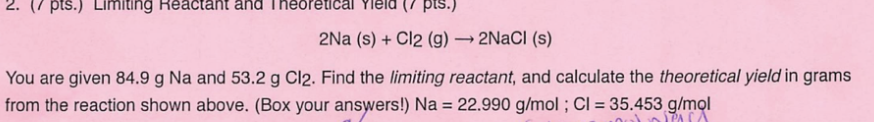2. ( pls.) Limiting Reactant and Theoretical Yield (7 pts.) 2Na (s) + Cl2 (g) → 2NaCl (s) You are given 84.9 g Na and 53.2 g Cl2. Find the limiting reactant, and calculate the theoretical yield in grams from the reaction shown above. (Box your answers!) Na = 22.990 g/mol; CI = 35.453 g/mol PACA

• ### 2. (7 pts.) Limiting Reactant and Theoretical Yield (7 pts.) 2Na (s) + Cl2 (g) -...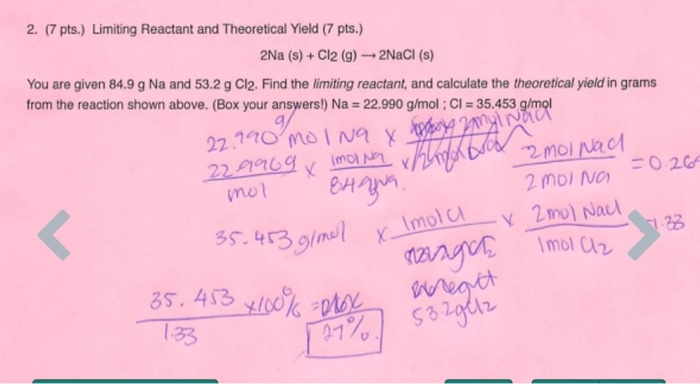2. (7 pts.) Limiting Reactant and Theoretical Yield (7 pts.) 2Na (s) + Cl2 (g) - 2NaCl (s) You are given 84.9 g Na and 53.2 g Cl2. Find the limiting reactant, and calculate the theoretical yield in grams from the reaction shown above. (Box your answers!) Na = 22.990 g/mol; CI = 35.453 g/mol 22.990 mo lng & Appoy angl nach 229909x imoina Trimorol mol Nad mol 8Hana 2 molNG =0.265 35.453 g/mol x Imola x 2 mol Nach...

• ### Balance the following equation:_____H_2(g)+_____N_2(g) rightarrow_____NH_3(g) Which is the limiting reagent, 4.43 moles of H_2 or 4.43...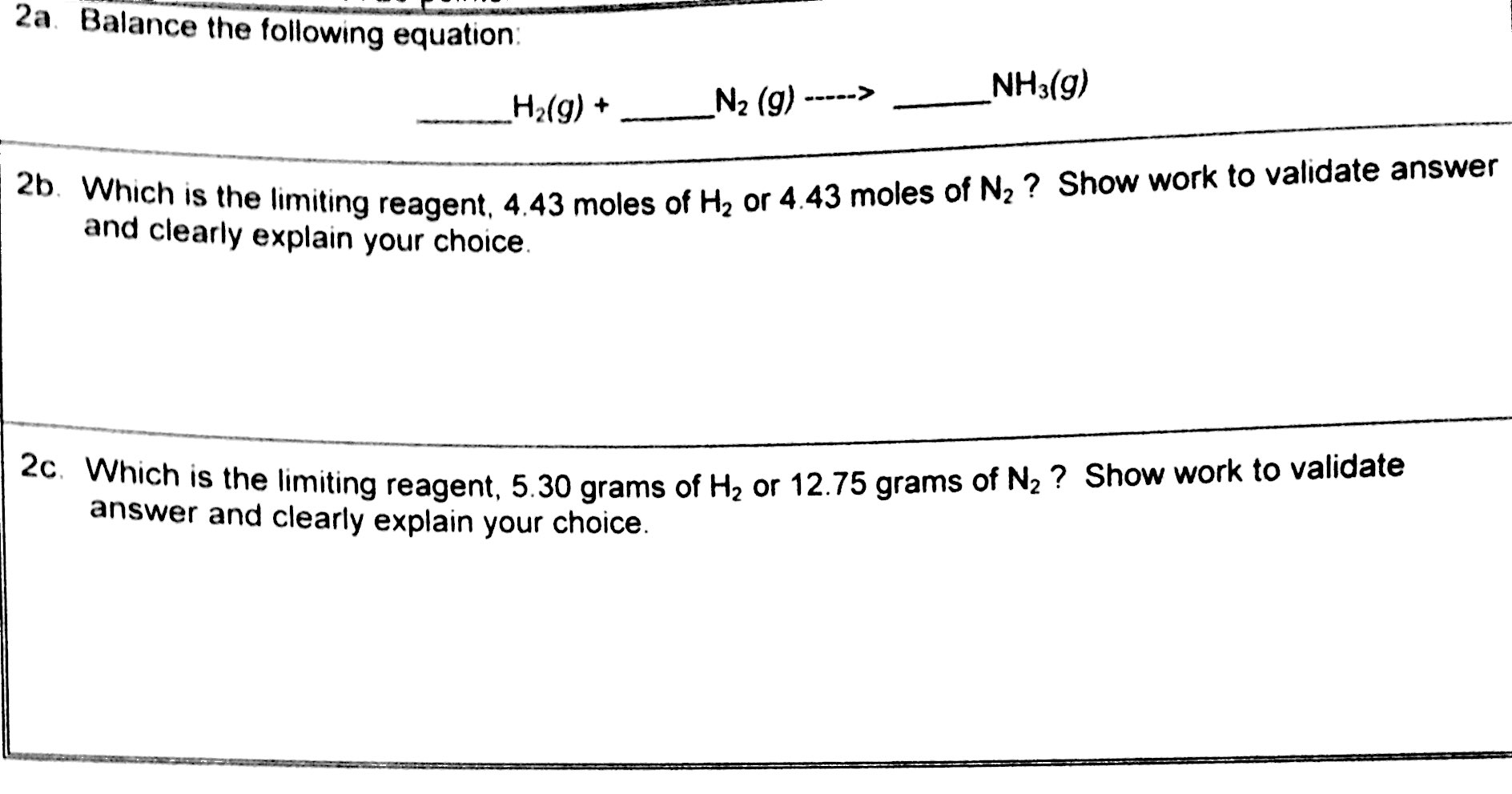Balance the following equation:_____H_2(g)+_____N_2(g) rightarrow_____NH_3(g) Which is the limiting reagent, 4.43 moles of H_2 or 4.43 moles of N_2? Show work to validate answer and clearly explain your choice. Which is the limiting reagent, 5.30 grams of H_2 or 12.75 grams of N_2? Show work to validate answer and clearly explain your choice.

• ### Consider the unbalanced equation: H2 + Cl2 = HClHow many grams of HCl can be produced if 5.00 g H2 are reacted with 125 g Cl2

Consider the unbalanced equation: H2 + Cl2 = HClHow many grams of HCl can be produced if 5.00 g H2 are reacted with 125 g Cl2?

• ### 7. What is a limiting reagent? The substance that is to when the chemical reaction that...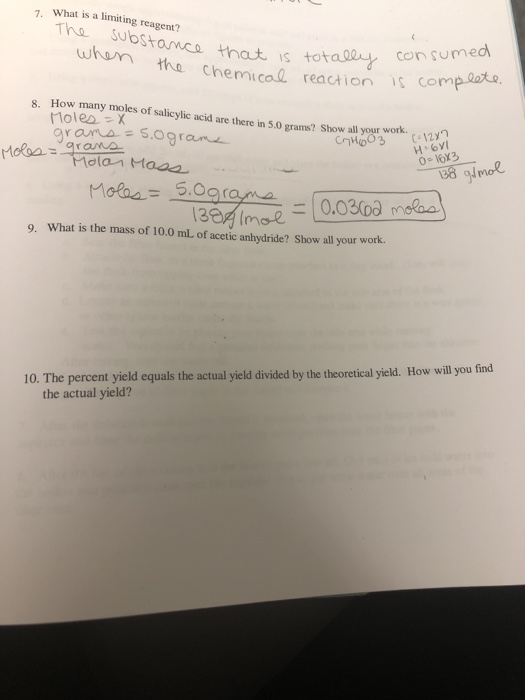7. What is a limiting reagent? The substance that is to when the chemical reaction that is totally hemical reaction consumed is complete. 8. How many moles of salicylic acid many moles of salicylic acid are there in 5.0 grams? Show all your work. Moles = x grams=5.ograma CyH603 (-128 moles 12 H6VI olor Moss 0 - 10X3 138 g/mol Moles= 5.ograme -0.0362 moles) 1389 Imolt 9. What is the mass of 10.0 mL of acetic anhydride? Show all your...

• ### Nitrogen and hydrogen react to form gaseous ammonia. a) Write the balanced chemical equation including states...

Nitrogen and hydrogen react to form gaseous ammonia. a) Write the balanced chemical equation including states of matter. b) If 50.0 g of nitrogen is mixed with 20.0 g of hydrogen what is the limiting reagent, show all work? c) What is the theoretical yield in grams of NH3? d) If the percent yield of the reaction is 62.0 % then what is the actual yield of the reaction?

• ### s References] TUTOR Limiting Reactants: Compare Reactant Moles The theoretical yield of a reaction is the...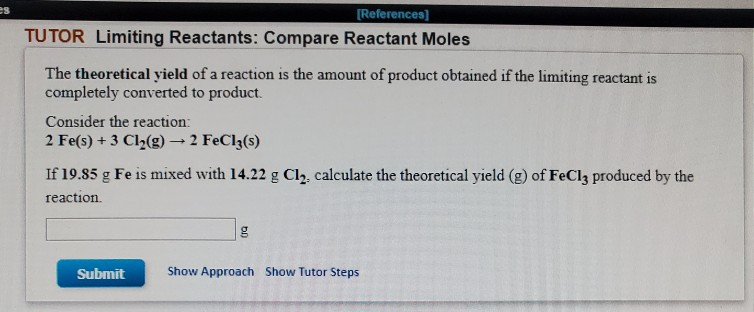s References] TUTOR Limiting Reactants: Compare Reactant Moles The theoretical yield of a reaction is the amount of product obtained if the limiting reactant is completely converted to product Consider the reaction: 2 Fe(s) +3 Cl2(g) 2 FeCl3(s) If 19.85 g Fe is mixed with 14.22 g Cl2, calculate the theoretical yield (g) of FeCl3 produced by the reaction g Show Approach Show Tutor Steps Submit